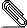# work factor calculation for brute-forcing crypto

```Hi folks,

Assume for a moment that we have a random number generator which is
non-uniform, and we are using it to generate a key.```
```
What I'd like to do is characterize the work factor involved in
brute-force search of the key space, assuming that the adversary
has knowledge of the characteristics of the random number generator?

The algorithm for this is simple:

Let the array X represent the probabilities of the outcomes of the
random number generator, sorted by probability, with x being the
probability of the most probable value.

Then, for a given fraction of the messages n (0 < n <= 1):

i = 0
m = 0
while (m + x[i]) < n:
m = m + x[i]
i = i + 1
return (i - 1) + (n - m) / (m + x[i])

This return value represents the average number of decryption attempts
required to guess the right key.  If one wanted to round up, one could
just return i instead of the last expression above, because the second
term is always in (0, 1]

I'm curious if there's a way to express this calculation as a
mathematical formula, rather than an algorithm, but right now I'm just
blanking on how I could do it.
--
Obama Nation | My emails do not have attachments; it's a digital signature
that your mail program doesn't understand. |
http://www.subspacefield.org/~travis/
If you are a spammer, please email j...@subspacefield.org to get blacklisted.
```pgpJ4gqi6vQJo.pgp
Description: PGP signature